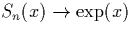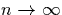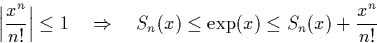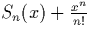Next: Natural Logarithm Function Up: Logarithmic Functions Previous: Logarithmic Functions

### Exponential Function

The exponential function is usually defined as follows:We can define a sequence S(x) such thatasas follows, using a second sequence s(x), whose current value is used to simplify the implementation:

If (x < 0), the sequence S oscillates about the limit. We can easily use two consecutive elements of the sequence to compute a lower and upper bound, and hence compute the required stream of nested intervals.

If (x > 0), the sequence no longer oscillates around the sequence, but tends to the limit from below. We can observe thatTherefore to create a sequence of nested intervals bounding the required limit, we simply find N such thatNow we simply compute nested intervals using the bounds above.

There is a problem with the above approach, which is that we cannot determine the sign of x without examining a potentially infinite number of digits, and yet we need to know the sign to determine the method to use. One solution to this is to `normalise' the input, but stopping after a finite number of steps if no non-zero digit is found. If we cannot determine the sign, we simply compute Sn-1, Sn, and, and then take the minimum and maximum of these numbers as lower and upper bounds respectively. This is the way the algorithm has been implemented in the calculator, and despite the extra cost, the method is very fast as both sequences converge extremely quickly for small values of |x|.Next: Natural Logarithm Function Up: Logarithmic Functions Previous: Logarithmic Functions
Martin Escardo
5/11/2000